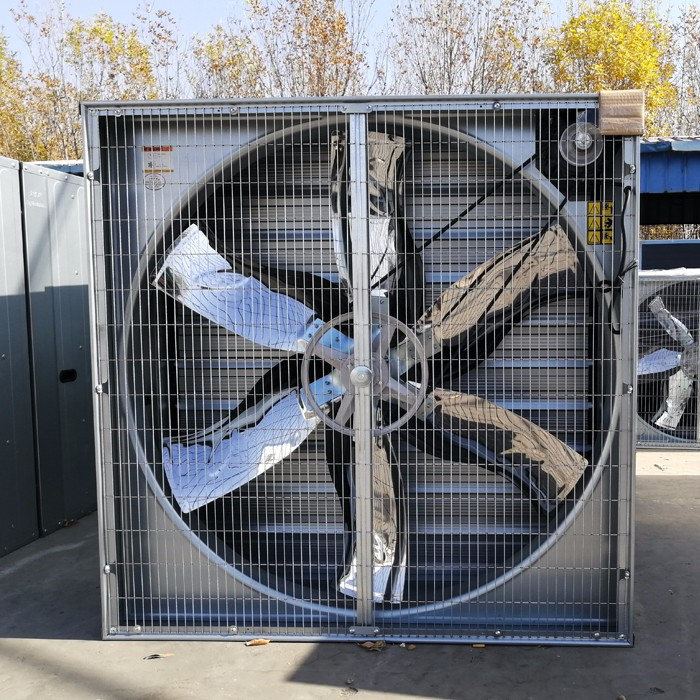# how to caculate max and min exhaust fans in broiler farm ventilation systemTo calculate the number of exhaust fans in broiler farm ventilation system, we will need some base data. Such as:

1.The poultry house dimension :

2.The air speed, which we will need inside the poultry house.

3. And the exhaust fan capacity.

For example, we know the dimension of poultry house L: 124m, W: 12m, H: 3.8m. And the wind across section area is : 13x3.8=45.6 m2.

And the air speed is 2.5m/s,

Max exhaust fan Capacity is 36000 m3/h,

and min exhaust fan capacity is : 16000m3/h.

Max:

According to above information, we will get to know that, the number of max fan is cross section area(45.6m2) x air speed (2.5m/s)x 60x60 (per hour) / capacity of max fan (16000m3/h).

That to calculate the max number of exhaust fan in number is :45.6x2.5x60x60 =410400m3/36000 = 11.4 = 12 fans.

That is: MAX need 12 exhaust fans with 36000m3/H for poultry broiler house.

MIN

If the min wind capacity is 1/4 than the max wind  capacity, then

And the same in Min in count:

Number of min fans is : Number of max fans(12) x capacity of max fan(36000) x 25%/16000 = 6.75 = 8 fans

Please feel free to give your inquiry in the form below. We will reply you in 24 hours.
Message